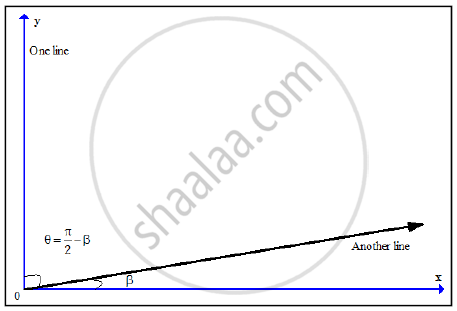HSC Science (Electronics) 12th Board ExamMaharashtra State Board
Share
Notifications

View all notifications
Books Shortlist
Your shortlist is empty

# If θ is the acute angle between the lines represented by equation ax2 + 2hxy + by2 = 0  then prove - HSC Science (Electronics) 12th Board Exam - Mathematics and Statistics

Login
Create free account

Forgot password?

#### Question

If θ is the acute angle between the lines represented by equation ax2 + 2hxy + by2 = 0  then prove that tantheta=|(2sqrt(h^2-ab))/(a+b)|, a+b!=0

#### Solution

Case (1)

Let m1 and m2 are the slopes of the lines representedby
the equation ax2+2hxy+by2=0,
then  m1 + m2 =-2h/b and m1m=a/b
If θ is the acute angle between the lines,
then tantheta=|(m_1-m_2)/(1+m_1m_2)|

"now " (m_1-m_2)^2=(m_1+m_2)^2-4m_1m_2

(m_1-m_2)^2=((-2h)/b)^2-4(a/b)

(m_1-m_2)^2=(4(h^2-ab))/b^2

|m_1-m_2|=|(2sqrt(h^2-ab))/b|

"similarly "1+m_1m_2=1+a/b=(a+b)/b

"substituting in "tantheta=|(m_1-m_2)/(1+m_1m_2)| , " we get "

tantheta=|((2-sqrt(h^2-ab))/b)/((a+b)/b)|

tantheta=|(2-sqrt(h^2-ab))/(a+b)| , "if "a+b!=0

Case (2)
If one of the lines is parallel to the y-axis then one of the slopes m1,m2, does not exist. As the line passes through the origin so one line parallel is the y-axis, it's equation is
x=0 and b=0
The other line is ax+2hy=0 whose slope tab beta=-a/(2h)∴ The acute angle between the pair of lines is pi/2-beta

therefore tantheta=|tan(pi/2-beta)|=|cot beta|=|(2h)/a|

"put " b=0 " in " tantheta=|(2sqrt(h^2-ab))/(a+b)|, " we get " tantheta=|(2h)/a|

"Hence "tantheta=|(2sqrt(h^2-ab))/(a+b)| " is valid in both the cases."

Is there an error in this question or solution?

#### APPEARS IN

2014-2015 (March) (with solutions)
Question 3.1.1 | 3.00 marks

#### Video TutorialsVIEW ALL 

Solution If θ is the acute angle between the lines represented by equation ax2 + 2hxy + by2 = 0  then prove Concept: Acute Angle Between the Lines.
S